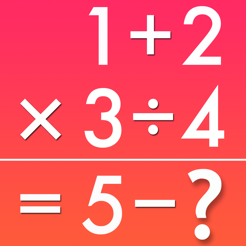## 截圖

•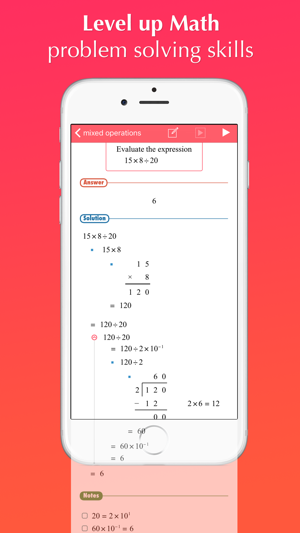•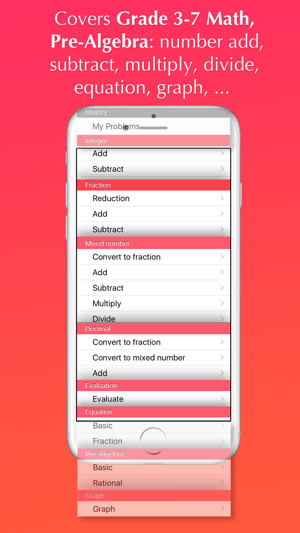•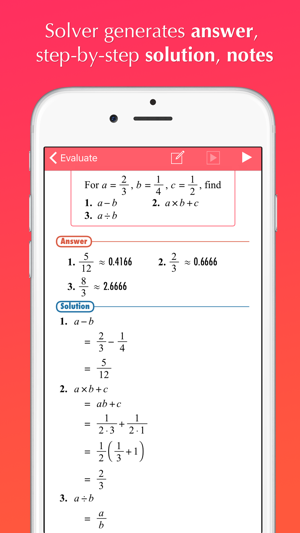•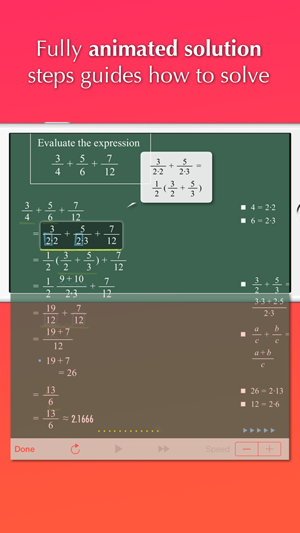•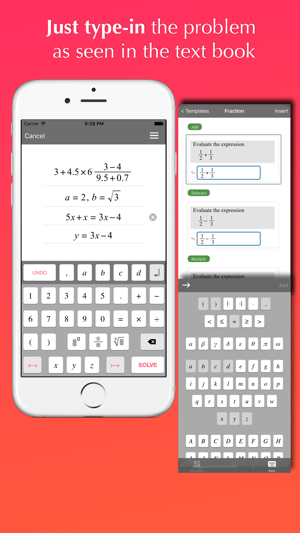## 簡介

Will guide you how to solve your math homework and textbook problems, anytime, anywhere.

FX Math Junior is a comprehensive math software, based on an automatic mathematical problem solving engine, including:

- Over 400 sample arithmetic problems and fully animated solution steps
- Automatic problem solving and generation of fully animated step-by-step procedures for problems typed in by users
- User friendly math problem expression editor (WYSIWYG mode)

FX Math Junior covers problems at the level of Grade 3-7 Math and Pre-Algebra courses:

- Number evaluations
- Prime factorization
- Fraction
- Mixed number
- Decimal
- Equation
- Graph

Bug fix

## 資訊

Euclidus Inc

39.7 MB

4+

App 內購買
1. FX Math Junior Premium Pack NT\$ 100

## 支援

•